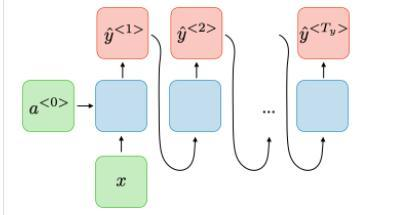# One to many LSTM

I’m looking for a way to implement one to many RNN/LSTM at PyTorch, but I can’t understand how to evaluate loss function and feed forward outputs of one hidden layer to another like at the pictureHere’s the raw LSTM code, could somebody help to adapt it?

``````class LSTMModel(nn.Module):
def __init__(self, input_dim, hidden_dim, layer_dim, output_dim):
super(LSTMModel, self).__init__()
# Hidden dimensions
self.hidden_dim = hidden_dim

# Number of hidden layers
self.layer_dim = layer_dim

# batch_first=True causes input/output tensors to be of shape
# (batch_dim, seq_dim, feature_dim)
self.lstm = nn.LSTM(input_dim, hidden_dim, layer_dim, batch_first=True)

self.fc = nn.Linear(hidden_dim, output_dim)
#self.fc2 = nn.Linear(hidden_dim, output_dim)

def forward(self, x):
# Initialize hidden state with zeros

out, (h_t, cn) = self.lstm(x, (h0, c0))
print(h_t.shape)
h_t=h_t.reshape([batch_size,layer_dim, hidden_dim])

out = self.fc(h_t[:, -1, :])

return out
``````

You may find the seq2seq tutorial useful, especially the decoder part.

``````class DecoderRNN(nn.Module):
def __init__(self, hidden_size, output_size):
super(DecoderRNN, self).__init__()
self.hidden_size = hidden_size

self.embedding = nn.Embedding(output_size, hidden_size)
self.gru = nn.GRU(hidden_size, hidden_size)
self.out = nn.Linear(hidden_size, output_size)
self.softmax = nn.LogSoftmax(dim=1)

def forward(self, input, hidden):
output = self.embedding(input).view(1, 1, -1)
output = F.relu(output)
output, hidden = self.gru(output, hidden)
output = self.softmax(self.out(output))
return output, hidden

def initHidden(self):
``````

Things to consider: the LSTM takes a hidden state and cell state. Its first input can be initialised as zeroes, as you do, but after that you have to provide the previous last hidden/cell state as the new input.

GRU is a good advice, thank you, I have all the y^i for the learning process, how should I calculate the loss?
And about LSTM, are there any options to do it via PyTorch, not implementing LSTM from scratch?

As loss you probably want to calculate NLLLoss after having done LogSoftMax on your logits (or you can have a look at CrossEntropyLoss).

``````criterion = nn.NLLLoss()
loss = criterion(output, target_tensor[i])
``````

where `i` is the i-th item that you are currently predicting.

In the code that I gave you can replace RU with LSTM. The difference in code being that GRU does not have a cell state, so it is easier to implement.

Finally, I did it in 2 ways:

1. One model

``````     optimizer.zero_grad()
layer[:, 0,:]=train.reshape(batch_size, input_dim).float().clone()

for i in range(1,time_dim):
layer[:, i,:] = model(layer[:, i-1,:].clone()).reshape(batch_size, input_dim).float()
loss[i-1]=error(layer[:, i,:].clone(), labels[:,i-1,:].clone())

loss=torch.sum(loss)
loss.backward()

optimizer.step()
``````
2. Many stacked models

``````     layer[:, 0,:]=train.reshape(batch_size, input_dim*2).float().clone()
for i in range(1,len(models)):
layer[:, i,:] = models[i-1](layer[:, i-1,:].clone()).reshape(batch_size, input_dim).float()
loss[i-1]=error(layer[:, i,:].clone(), labels[:,i-1:i,:].clone()

loss=torch.sum(loss)

loss.backward()

optimizer.step()
``````

Is there something, I could miss?

Did this worked properly?